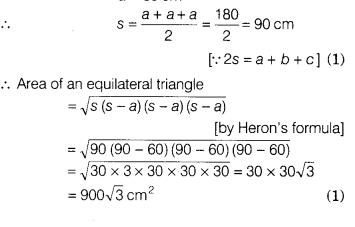# A traffic signal board, indicating ‘SCHOOL AHEAD’, is an equilateral triangle with side

A traffic signal board, indicating ‘SCHOOL AHEAD’, is an equilateral triangle with side a. Find the area of the signal board, using Heron’s formula.If its perimeter is 180 cm, what will be the area of the signal board?

We know that, an equilateral triangle has equal sides.
So, all sides are equal to a.
Perimeter of triangle = 180 cm [given]
=> a + a + a = 180
3a = 180
a = 60 cm
strong text